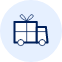MAI SUNT 00:00:00:00

MAI SUNT

X# Concepts of Modern Mathematics

## De (autor): Ian StewartIn this charming volume, a noted English mathematician uses humor and anecdote to illuminate the concepts underlying "new math"--groups, sets, subsets, topology, Boolean algebra, and more. By the time readers finish this book, they shall have a much clearer grasp of how modern mathematicians look at figures, function, and formulas, leading them to a better comprehension of the nature of the mathematics itself.

Some years ago, new math took the country's classrooms by storm. Based on the abstract, general style of mathematical exposition favored by research mathematicians, its goal was to teach students not just to manipulate numbers and formulas, but to grasp the underlying mathematical concepts. The result, at least at first, was a great deal of confusion among teachers, students, and parents. Since then, the negative aspects of new math have been eliminated and its positive elements assimilated into classroom instruction.
In this charming volume, a noted English mathematician uses humor and anecdote to illuminate the concepts underlying new math: groups, sets, subsets, topology, Boolean algebra, and more. According to Professor Stewart, an understanding of these concepts offers the best route to grasping the true nature of mathematics, in particular the power, beauty, and utility of pure mathematics. No advanced mathematical background is needed (a smattering of algebra, geometry, and trigonometry is helpful) to follow the author's lucid and thought-provoking discussions of such topics as functions, symmetry, axiomatics, counting, topology, hyperspace, linear algebra, real analysis, probability, computers, applications of modern mathematics, and much more.
By the time readers have finished this book, they'll have a much clearer grasp of how modern mathematicians look at figures, functions, and formulas and how a firm grasp of the ideas underlying new math leads toward a genuine comprehension of the nature of mathematics itself.

Citeste mai mult

-10%

transport gratuit

79.43Lei

88.26 Lei

Sau 7943 de puncteFiecare comanda noua reprezinta o investitie pentru viitoarele tale comenzi. Orice comanda plasata de pe un cont de utilizator primeste in schimb un numar de puncte de fidelitate, In conformitate cu regulile de conversiune stabilite. Punctele acumulate sunt incarcate automat in contul tau si pot fi folosite ulterior, pentru plata urmatoarelor comenzi.

Livrare in 2-4 saptamaniRidicare Personala GRATUITA!

easybox / Ship&Go TOATA luna August!

#### Descrierea produsului

In this charming volume, a noted English mathematician uses humor and anecdote to illuminate the concepts underlying "new math"--groups, sets, subsets, topology, Boolean algebra, and more. By the time readers finish this book, they shall have a much clearer grasp of how modern mathematicians look at figures, function, and formulas, leading them to a better comprehension of the nature of the mathematics itself.

Some years ago, new math took the country's classrooms by storm. Based on the abstract, general style of mathematical exposition favored by research mathematicians, its goal was to teach students not just to manipulate numbers and formulas, but to grasp the underlying mathematical concepts. The result, at least at first, was a great deal of confusion among teachers, students, and parents. Since then, the negative aspects of new math have been eliminated and its positive elements assimilated into classroom instruction.
In this charming volume, a noted English mathematician uses humor and anecdote to illuminate the concepts underlying new math: groups, sets, subsets, topology, Boolean algebra, and more. According to Professor Stewart, an understanding of these concepts offers the best route to grasping the true nature of mathematics, in particular the power, beauty, and utility of pure mathematics. No advanced mathematical background is needed (a smattering of algebra, geometry, and trigonometry is helpful) to follow the author's lucid and thought-provoking discussions of such topics as functions, symmetry, axiomatics, counting, topology, hyperspace, linear algebra, real analysis, probability, computers, applications of modern mathematics, and much more.
By the time readers have finished this book, they'll have a much clearer grasp of how modern mathematicians look at figures, functions, and formulas and how a firm grasp of the ideas underlying new math leads toward a genuine comprehension of the nature of mathematics itself.

Citeste mai mult

Detaliile produsului

## De acelasi autor

Parerea ta e inspiratie pentru comunitatea Libris!

Noi suntem despre carti, si la fel este si